sitemap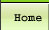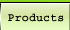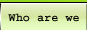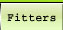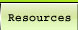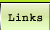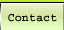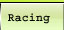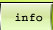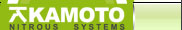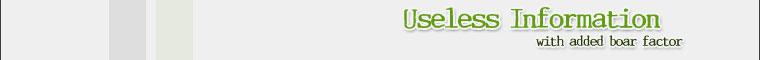What is Torque ?

Torque is a force that tends to rotate or turn things. You generate a torque any time you apply a force using a wrench. Tightening the lug nuts on your wheels is a good example. When you use a wrench, you apply a force to the handle. This force creates a torque on the lug nut, which tends to turn the lug nut.

English units of torque are pound-inches or pound-feet; the SI unit is the Newton-meter. Notice that the torque units contain a distance and a force. To calculate the torque, you just multiply the force by the distance from the center. In the case of the lug nuts, if the wrench is a foot long, and you put 200 pounds of force on it, you are generating 200 pound-feet of torque. If you use a 2-foot wrench, you only need to put 100 pounds of force on it to generate the same torque.
A car engine creates torque and uses it to spin the crankshaft. This torque is created exactly the same way: A force is applied at a distance. Let's take a close look at some of the engine parts:

The combustion of gas in the cylinder creates pressure against the piston. That pressure creates a force on the piston, which pushes it down. The force is transmitted from the piston to the connecting rod, and from the connecting rod into the crankshaft. In Figure 2, notice that the point where the connecting rod attaches to the crank shaft is some distance from the center of the shaft. The horizontal distance changes as the crankshaft spins, so the torque also changes, since torque equals force multiplied by distance.

You might be wondering why only the horizontal distance is important in determining the torque in this engine. You can see in Figure 2 that when the piston is at the top of its stroke, the connecting rod points straight down at the center of the crankshaft. No torque is generated in this position, because only the force that acts on the lever in a direction perpendicular to the lever generates a torque.

If you have ever tried to loosen really tight lug nuts on your car, you know a good way to make a lot of torque is to position the wrench so that it is horizontal, and then stand on the end of the wrench -- this way you are applying all of your weight at a distance equal to the length of the wrench. If you were to position the wrench with the handle pointing straight up, and then stand on the top of the handle (assuming you could keep your balance), you would have no chance of loosening the lug nut. You might as well stand directly on the lug nut.

Figure 3 shows the maximum torque and power generated by two different engines. One engine is a turbocharged Caterpillar C-12 diesel truck engine. This engine weighs about 2,000 pounds, and has a displacement of 732 cubic inches (12 liters). The other engine is a highly modified Ford Mustang Cobra engine, with a displacement of 280 cubic inches (4.6 liters); it has an added supercharger and weighs about 400 pounds. They both produce a maximum of about 430 horsepower (hp), but only one of these engines is suitable for pulling a heavy truck. The reason lies partly in the power/torque curve shown above.
When the animation pauses, you can see that the Caterpillar engine produces 1,650 lb-ft of torque at 1200 rpm, which is 377 hp. At 5,600 rpm, the Mustang engine also makes 377 hp, but it only makes 354 lb-ft of torque. If you have read the article on gear ratios, you might be thinking of a way to help the Mustang engine produce the same 1,650 lb-ft of torque. If you put a gear reduction of 4.66:1 on the Mustang engine, the output speed would be (5,600/4.66 rpm) 1,200 rpm, and the torque would be (4.66 * 354 lb-ft) 1,650 lb-ft -- exactly the same as the big Caterpillar engine.

Now you might be wondering, why don't big trucks use small gas engines instead of big diesel engines? In the scenario above, the big Caterpillar engine is loafing along at 1,200 rpm, nice and slow, producing 377 horsepower. Meanwhile, the small gas engine is screaming along at 5,600 rpm. The small gas engine is not going to last very long at that speed and power output. The big truck engine is designed to last years, and to drive hundreds of thousands of miles each year it lasts.

What is Work?
The work we are talking about here is work in the physics sense. Not home work, or chores, or your job or any other type of work. It is good old mechanical work. Work is simply the application of a force over a distance, with one catch -- the distance only counts if it is in the direction of the force you apply. Lifting a weight from the ground and putting it on a shelf is a good example of work. The force is equal to the weight of the object, and the distance is equal to the height of the shelf. If the weight were in another room, and you had to pick it up and walk across the room before you put it on the shelf, you didn't do any more work than if the weight were sitting on the ground directly beneath the shelf. It may have felt like you did more work, but while you were walking with the weight you moved horizontally, while the force from the weight was vertical. Your car also does work. When it is moving, it has to apply a force to counter the forces of friction and aerodynamic drag. If it drives up a hill, it does the same kind of work that you do when lifting a weight. When it drives back down the hill, however, it gets back the work it did. The hill helps the car drive down.
Work is energy that has been used. When you do work, you use energy. But sometimes the energy you use can be recovered. When the car drives up the hill, the work it does to get to the top helps it get back down. Work and energy are closely related. The units of work are the same as the units of energy, which we will discuss later.

Power is a measure of how quickly work can be done. Using a lever, you may be able to generate 200 ft-lb of torque. But could you spin that lever 3,000 times per minute? That is exactly what your car engine does. The SI unit for power is the watt. A watt breaks down into other units that we have already talked about. One watt is equal to 1 Newton-meter per second (Nm/s). You can multiply the amount of torque in Newton-meters by the rotational speed in order to find the power in watts. Another way to look at power is as a unit of speed (m/s) combined with a unit of force (N). If you were pushing on something with a force of 1 N, and it moved at a speed of 1 m/s, your power output would be 1 watt. An interesting way to figure out how much power you can output is to see how quickly you can run up a flight of stairs.

1. Measure the height of a set of stairs that takes you up about three stories.
2. Time yourself while you run up the stairs as quickly as possible.
3. Divide the height of the stairs by the time it took you to ascend them. This will give you your speed.
For instance, if it took you 15 seconds to run up 10 meters, then your speed was 0.66 m/s (only your speed in the vertical direction is important). Now you need to figure out how much force you exerted over those 10 meters, and since the only thing you hauled up the stairs was yourself, this force is equal to your weight. To get the amount of power you output, multiply your weight by your speed.
Power (W) = (height of stairs (m) / Time to climb (s) ) * weight (N)
Power (hp) = [(height of stairs (ft) / Time to climb (s) ) * weight (lb)] / 550

Energy is the final chapter in our terminology saga. We'll need everything we've learned up to this point to explain energy.
If power is like the strength of a weightlifter, energy is like his endurance. Energy is a measure of how long we can sustain the output of power, or how much work we can do. Power is the rate at which we do the work. One common unit of energy is the kilowatt-hour (kWh). You learned in the last section that a kW is a unit of power. If we are using one kW of power, a kWh of energy will last one hour. If we use 10 kW of power, we will use up the kWh in just six minutes.
There are two kinds of energy: potential and kinetic.

Potential Energy
Potential energy is waiting to be converted into power. Gasoline in a fuel tank, food in your stomach, a compressed spring, and a weight hanging from a tree are all examples of potential energy.
The human body is a type of energy-conversion device. It converts food into power, which can be used to do work. A car engine converts gasoline into power, which can also be used to do work. A pendulum clock is a device that uses the energy stored in hanging weights to do work.

When you lift an object higher, it gains potential energy. The higher you lift it, and the heavier it is, the more energy it gains. For example, if you lift a bowling ball 1 inch, and drop it on the roof of your car, it won't do much damage (please, don't try this). But if you lift the ball 100 feet and drop it on your car, it will put a huge dent in the roof. The same ball dropped from a greater height has much more energy. So, by increasing the height of an object, you increase its potential energy.
Let's go back to our experiment in which we ran up the stairs and found out how much power we used. There is another way to look at how we calculated our power: We calculated how much potential energy our body gained when we raised it up to a certain height. This amount of energy was the work we did by running up the stairs (force * distance, or our weight * the height of the stairs). We then calculated how long it took to do this work, and that's how we found out the power. Remember that power is the rate at which we do work.

The formula to calculate the potential energy (PE) you gain when you increase your height is:
PE = Force * Distance
In this case, the force is equal to your weight, which is your mass (m) * the acceleration of gravity (g), and the distance is equal to your height (h) change. So the formula can be written:
PE = mgh
Kinetic Energy
Kinetic energy is energy of motion. Objects that are moving, such as a roller coaster, have kinetic energy (KE). If a car crashes into a wall at 5 mph, it shouldn't do much damage to the car. But if it hits the wall at 40 mph, the car will most likely be totaled.
Kinetic energy is similar to potential energy. The more the object weighs, and the faster it is moving, the more kinetic energy it has. The formula for KE is:
KE = 1/2*m*v2,
where m is the mass and v is the velocity.
One of the interesting things about kinetic energy is that it increases with the velocity squared. This means that if a car is going twice as fast, it has four times the energy. You may have noticed that your car accelerates much faster from 0 mph to 20 mph than it does from 40 mph to 60 mph. Let's compare how much kinetic energy is required at each of these speeds. At first glance, you might say that in each case, the car is increasing its speed by 20 mph, and so the energy required for each increase must be the same. But this is not so.

We can calculate the kinetic energy required to go from 0 mph to 20 mph by calculating the KE at 20 mph and then subtracting the KE at 0 mph from that number. In this case, it would be 1/2*m*202 - 1/2*m*02. Because the second part of the equation is 0, the KE = 1/2*m*202, or 200 m. For the car going from 40 mph to 60 mph, the KE = 1/2*m*602 - 1/2*m*402; so KE = 1,800 m - 800 m, or 1000 m. Comparing the two results, we can see that it takes a KE of 1,000 m to go from 40 mph to 60 mph, whereas it only takes 200 m to go from 0 mph to 20 mph.
There are a lot of other factors involved in determining a car's acceleration, such as aerodynamic drag, which also increases with the velocity squared. Gear ratios determine how much of the engine's power is available at a particular speed, and traction is sometimes a limiting factor. So it's a lot more complicated than just doing a kinetic energy calculation, but that calculation does help to explain the difference in acceleration times.

Bringing it Together
Now that we know about potential energy and kinetic energy, we can do some interesting calculations. Let's figure out how high a pole-vaulter could jump if he had perfect technique. First we'll figure out his KE, and then we'll calculate how high he could vault if he used all of that KE to increase his height (and therefore his PE), without wasting any of it. If he converted all of his KE to PE, then we can solve the equation by setting them equal to each other:
1/2*m*v2 = m*g*h
Since mass is on both sides of the equation, we can eliminate this term. This makes sense because both KE and PE increase with increasing mass, so if the runner is heavier, his PE and KE both increase. So we'll eliminate the mass term and rearrange things a little to solve for h:
1/2*v2 / g = h
Let's say our pole-vaulter can run as fast as anyone in the world. Right now, the world record for running 100 m is just under 10 seconds. That gives a velocity of 10 m/s. We also know that the acceleration due to gravity is 9.8 m/s2. So now we can solve for the height:
1/2*10 / 9.8 = 5.1 meters
So 5.1 meters is the height that a pole-vaulter could raise his center of mass if he converted all of his KE into PE. But his center of mass is not on the ground; it is in the middle of his body, about 1 meter off the ground. So the best height a pole-vaulter could achieve is in fact about 6.1 meters, or 20 feet. He may be able to gain a little more height by using special techniques, like pushing off from the top of the pole, or getting a really good jump before takeoff.
In Figure 4 you can see how the pole-vaulter's energy changes as he makes the vault. When he starts out, both his potential and kinetic energy are zero. As he starts to run, he increases his kinetic energy. Then, as he plants the pole and starts his vault, he trades his kinetic energy for potential energy. As the pole bends, it absorbs a lot of his kinetic energy, just like compressing a spring. He then uses the potential energy stored in the pole to raise his body over the bar. At the top of his vault, he has converted most of his kinetic energy into potential energy.
Our calculation compares pretty well with the current world record of 6.15 meters, set by Sergey Bubka in 1993.

 Akamoto offers a great range of Performance Parts including Alloy Wheels, Body Kits, Performance Parts, Exhausts, Street Racing, Drag Racing, Superchargers, Nitrous Oxide, Drifting, Car Tuning, Turbo chargers.

© Copyright 2001 - 2009 Akamoto.co.uk All rights reserved - Website design and maintenance by Dualmedia.co.uk a Web design and Search engine marketing company